Detalles de la revistaFormato
Revista
eISSN
2300-0929
Primera edición
19 Oct 2012
Calendario de la edición
4 veces al año
Idiomas
InglésAcceso abierto

# Effect of Different Yarn Combinations on Auxetic Properties of Plied Yarns

###### Páginas: -
Detalles de la revistaFormato
Revista
eISSN
2300-0929
Primera edición
19 Oct 2012
Calendario de la edición
4 veces al año
Idiomas
Inglés

This study presents the effects of a novel plied yarn structure consisting of different yarn components and yarn twist levels on the Poisson's ratio and auxetic behavior of yarns. The plied yarn structures are formed with bulky and soft yarn components (helical plied yarn [HPY], braided yarn, and monofilament latex yarn) and stiff yarn components (such as high tenacity [HT] and polyvinyl chloride [PVC]-coated polyester yarns) to achieve auxetic behavior. Experimental results showed that as the level of yarn twist increased, the Poisson's ratios and the tensile modulus values of the plied yarns decreased, but the elongation values increased. A negative Poisson's ratio (NPR) was obtained in HT–latex and PVC–latex plied yarns with a low twist level. The plied yarns formed with braid–HPY and braid–braid components gave partial NPR under tension. A similar result was achieved for yarns with HT–latex and PVC–latex components. Since partial NPR was seen in novel plied yarns with braided and HPY components, it is concluded that yarns formed with bulky–bulky yarn components could give an auxetic performance under tension.

#### Keywords

Introduction

Auxetic materials have a negative Poisson's ratio (NPR). They expand in the lateral direction under a longitudinal tensile strength and laterally contract when subjected to compressive strength [1, 2].

In literature, the auxetic yarn structures that have been reported are the helical structure, semiauxetic structure, and plied yarn structure [3,4,5,6,7,8,9,10,11,12]. The helical auxetic yarn (HAY) structure was first presented by Hook [11, 13]. The HAY structure is formed by combining two yarn components with different stiffness levels in a double-helical form. The HAY structure consists of a straight elastomeric yarn called as a “core” and a relatively stiff yarn structure that is wrapped around this core yarn (Figure 1). Under tensile loading, the stiff wrap yarn is straightened and displaces the core yarn into a curved shape; as a result of this, the yarn structure is expanded in the lateral direction [11, 13].

A study on the HAY structure showed that the starting angle of the wrap fiber determined the magnitude of auxetic behavior . The auxetic performance was also affected by the diameter ratio of wrap-to-core fibers and these fibers’ native Poisson's ratios . In another study on auxetic yarns, it was found that the NPR effect became more noticeable with a higher diameter ratio and lower helical angle, as well as a larger tensile modulus of the wrap filament . In another study, a novel auxetic plied yarn structure showed an NPR effect under axial extension. However, it was stated that the auxetic effect varied with axial strain .

For the Poisson's ratio of HAYs, the diameters, the ratio of the elastic moduli of the core and wrap yarns, and the initial wrap angle parameters were investigated [5, 6, 12]. It was found that the HAY structure had some limitations. In the HAY structure, the stiff wrap yarn could easily slip along the surface of the core yarn under repeated extension. Besides, difficulties might arise if the yarn twist process is not completed properly. Since the stiff wrap yarn could easily become loose after extension, this could cause a decrease in the structural stability of the yarn [3,4,5, 11,12,13].

Liu et al.  reported a novel interlaced-helical wrapping yarn with NPR and a stable structure. The differences in geometry and auxetic behavior between helical and interlaced-helical yarn structures were analyzed. Results showed that the interlaced-helical complex yarn has advantages over a helical wrap yarn with the same materials and initial wrap angle in terms of both structural stability and auxetic effect. The results also showed that all interlaced-helical wrap yarns with lower initial wrap angle and larger tensile modulus of the stiff yarns would produce a better auxetic effect .

Jiang and Hu  reported a new type of auxetic yarn made with circular braiding technology to overcome the yarn slippage problem in the conventional HAY structure. Results showed that the slippage problem was eliminated in the newly developed auxiliary yarn structure; moreover, early activation and a higher magnitude of NPR could be achieved. The results also showed that all structural parameters, including stiff yarn number and arrangement, core yarn, and elastic yarn diameter, influence the auxetic effect of the novel yarn structure .

A novel type of braided structure with NPR behavior was proposed in a study on the tubular braided structure. It was found that the NPR behavior could be achieved in a specially designed tubular braided structure with the use of a wrap yarn having a higher modulus than the braiding yarns and core yarn. It was shown that the effects of three structural parameters, such as the initial wrap angle, the initial braiding angle, and the braiding yarn diameter, influenced the tensile behaviors and the NPR effect of the auxetic braided structure. It was found that the wrap angle had more noticeable influences than the initial braiding angle and the braiding yarn diameter. It was concluded that an auxetic braided structure with a lower initial wrap angle, a higher initial braiding angle, and a larger braiding yarn diameter had a better auxetic performance .

In this study, the effects of a novel plied yarn structure, consisting of different yarn components and yarn twist levels, on the Poisson's ratio and auxetic behavior of yarns were examined. In particular, it was investigated whether the plied yarn structures formed by bulky yarn components, such as braid and helical plied yarn (HPY), showed changes in the Poisson's ratio under tension and a possible auxetic behavior.

Experimental
Materials

In this study, the tension-related auxetic effect of novel plied yarns at different twist levels (100, 200, and 300 T/m) formed by yarn components with different structural properties has been evaluated. The rotating probe of the Officine Brustio test device (Figure 2) was used to twist the two component yarns together to form the novel plied yarn structure. The yarn components to be combined were attached between the jaws and twisted to give the specified number of twists per unit length.

The structural properties and the tensile strength values of the component yarns are given in Tables 1 and 2, respectively. In Table 3, the codes of the novel plied yarn and the tensile strength properties at twist levels of 100, 200, and 300 T/m are given.

Component yarn properties.

Component yarnYarn propertiesYarn count (tex)
HTHigh tenacity polyester9.444
HPYHelical plied yarn8 pieces of 33.333/96 tex/filament textured polyester yarn were produced by folding them by twisting at 70 T/m.306.333
BraidCircular braided yarn with 8 carriers.(4 × 33.333/96 tex/filament textured polyester + 4 × 9.444/36 tex/filament HT polyester)(The braided yarn was produced by attaching to the carrier in a 1:1 layout.)194.222
PVCPVC-coated polyester111.111
LatexMonofilament latex250.000

HPY, helical plied yarn; HT, high tenacity; PVC, polyvinyl chloride.

Tenacity and elongation values of component yarns.

Component yarnTenacity (N/tex)Maximum elongation (%)
HT0.6513.60
HPY0.3026.80
Braid0.3219.17
PVC0.1417.60

HPY, helical plied yarn; HT, high tenacity; PVC, polyvinyl chloride.

Properties of the novel plied yarn.

Yarn codeYarn count (tex)Yarn componentsYarn twist (T/m)Tenacity (N/tex)Maximum elongation (%)
A315.777HPY–HT1000.2913.66
2000.2837.02
3000.2842.77
B500.555HPY–braid1000.2827.76
2000.2836.92
3000.2536.50
C388.444Braid–braid1000.2918.39
2000.2823.03
3000.2626.53
D259.444HT–latex1000.02513.19
2000.02213.49
3000.02314.22
E361.111PVC–latex1000.04613.51
2000.04313.86
3000.04314.55
F305.333Braid–PVC1000.2214.92
2000.2318.35
3000.2022.91
G444.222Braid–latex1000.1318.93
2000.1220.64
3000.1224.47

HPY, helical plied yarn; HT, high tenacity; PVC, polyvinyl chloride.

Methods
Tensile testing and calculation of Poisson's ratio

The tensile tests were carried out on a Shimadzu AG-X plus testing machine. The gage length and tensile test speed were set at 250 mm and 10 mm/min, respectively. To calculate the radial strain of the yarn structure for any given axial strain, the yarns were photographed at 50 times magnification using a digital microscope (INSIZE ISM-PRO) with a time interval of 5 sec (which corresponded to a 0.83 mm extension), until an elongation of 30 mm was obtained during the tensile testing. The setup of the testing system is presented in Figure 3.

The average yarn diameter was calculated by a software developed using MatLab, which takes the average of 1,200 readings of the diameter through the length of a 10 mm yarn. Moreover, for each yarn, this measurement was taken from both the middle and the bottom regions of the yarn held by the clamp of the device. It was seen that the yarn diameter values obtained from the two regions were close to each other. Based on this result, the yarn structure was evaluated as homogeneous. Considering that the yarn consists of a homogeneous and continuous structure, it can be said that the elongation reflects equally on each region of the yarn. The average values of the results obtained from two different regions were taken as the yarn diameter in the evaluations.

In addition, the yarn diameters were measured manually with the help of ImageJ program on the yarn images. Correlation coefficients between the yarn diameter values obtained from ImageJ and the results obtained from MatLab were calculated. Correlation coefficient values between the results obtained by the two measurement methods were in the range of 0.9966–0.9991.

A digital microscope was utilized to capture images of the yarns at a 10 mm distance. Subsequently, thresholding operation was applied to the images using MatLab to create binary images and obtain the outer edges of the yarns. A computer program developed to obtain the yarn diameter processed the binary image in the following order: the number of pixels was counted starting from one side of the yarn edge to the other side. This operation was applied for each row in the binary image along the yarn. Then, the average number of pixels between the outer edges was calculated. Finally, the average pixel value was converted to millimeter scale using the known image dimensions in millimeters to obtain the average yarn diameter.

D0 and D represented the diameters at the initial and the stretched states of the yarns, respectively. The radial strain Ɛr was calculated from Eq. (1). As the axial strain (Ɛa) was directly provided by the tensile tester, the Poisson's ratio, n, of the plied yarn structure ν was calculated using Eq. (2) [11, 12].

$Ɛr=(D/D0−1)×100%${\varepsilon _r} = \left( {D/{D_{\it 0}} - 1} \right) \times 100\% $ν=−Ɛr/Ɛa$\nu = - {\varepsilon _r}/{\varepsilon _a}

The binary images of the yarns produced at different twist levels (50 times magnification) are presented under different tension values, such as tension-free state, 5 sec (0.83 mm elongation), 10 sec (1.67 mm elongation), and 60 sec (10 mm elongation).

Results and discussion
Assessment of the changes in Poisson's ratios of the plied yarns under different elongations

The Poisson's ratios for 10, 20, and 30 mm elongations depending on the different twist levels of novel plied yarns are presented in Figures 4–6.Changes in Poisson's ratios of yarns at 10 mm elongation.HPY, helical plied yarn; HT, high tenacity; PVC, polyvinyl chloride.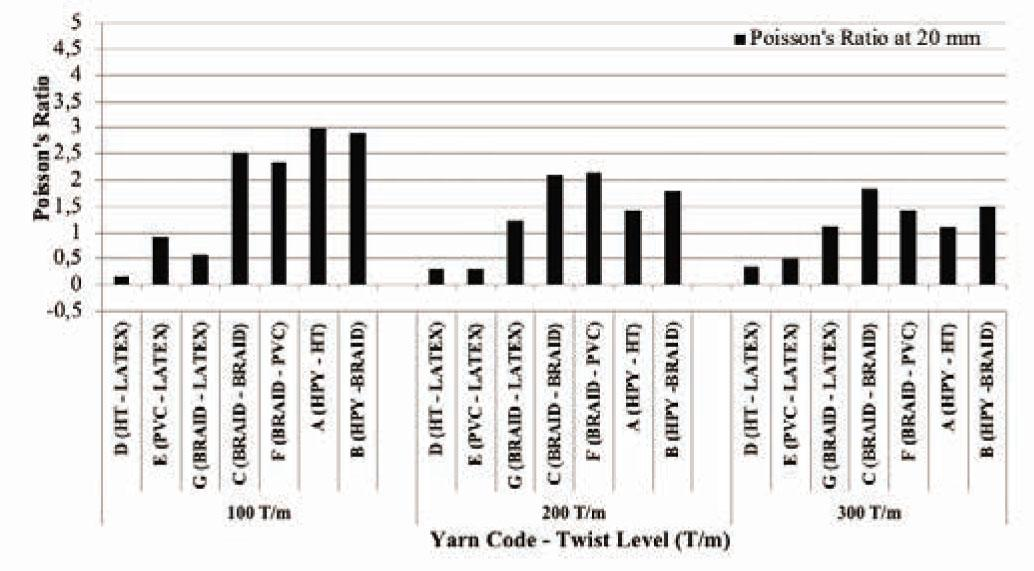Changes in Poisson's ratios of yarns at 20 mm elongation.HPY, helical plied yarn; HT, high tenacity; PVC, polyvinyl chloride.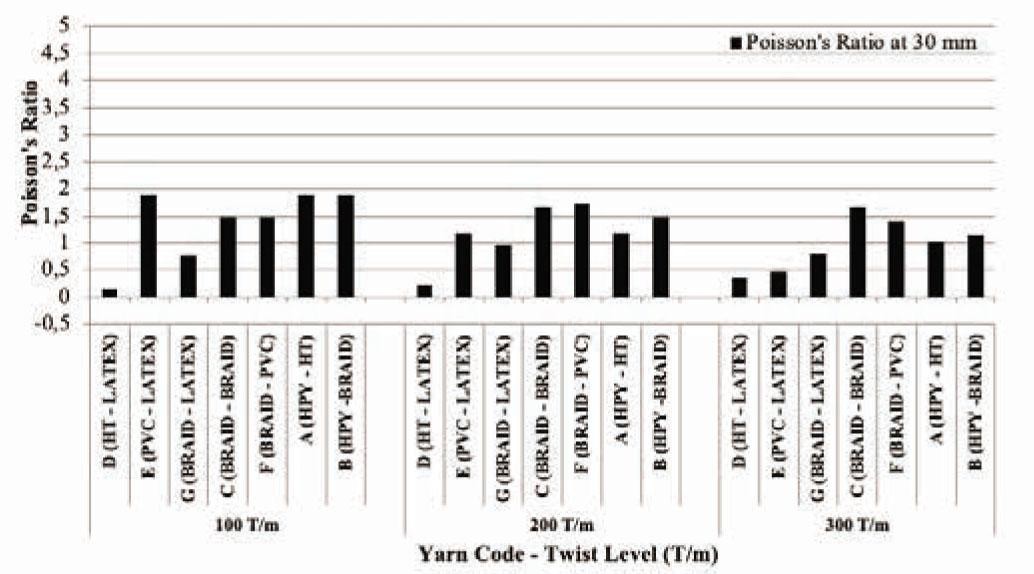Changes in Poisson's ratios of yarns at 30 mm elongation.HPY, helical plied yarn; HT, high tenacity; PVC, polyvinyl chloride.

In Figure 4, it was observed that for novel plied yarn structures containing a monofilament latex component, NPRs were obtained at a low twist level (100 T/m). This result was obtained if the yarn that combined with latex, as in D and E yarns, were stiff component yarns, such as HT and polyvinyl chloride (PVC) polyester. While it was observed that the yarn structure formed with HT and PVC yarns with latex components had an NPR of 100 T/m, the Poisson's ratio was positive as the twist level was increased. In the latex–braid component yarn structure (G), NPR could not be obtained. However, it was seen that the G yarn with low twist level gave a low Poisson's ratio at 10 mm elongation compared to other yarns.

In Figure 4, for the D, E, and G yarn structures, it was seen that as the yarn twist level increased, the values of Poisson's ratio increased. However, in the other yarn groups (C, F, A, and B), as the twist level increased, the Poisson's ratio of the yarns decreased significantly. When the properties of C, F, A, and B yarns were examined (Table 3), it was observed that these yarn groups have a bulky multifilament structure such as braid and HPY. Especially, in the C yarn (braid–braid), it was found that the Poisson's ratio approached ≈ 0 at high twist levels such as 300 T/m.

In the F (braid–PVC), A (HPY–HT), and B (HPY–braid) yarns with 100 T/m, the Poisson's ratios were around 3.5–4.5, while the values dropped to about 1.5 at 300 T/m twist level. Furthermore, bulky–stiff (F and A) and bulky–bulky (B) yarn structures were found to give approximately the same Poisson's ratios at high twist value (300 T/m).

The C (braid–braid; bulky–bulky) and D (HT–latex; stiff–bulky) yarn structures gave Poisson's ratio values close to each other at 300 T/m twist level. Similarly, it was observed that the E (PVC–latex; stiff–bulky), F (braid–PVC; bulky–stiff), A (HPY–HT; bulky–stiff), and B (HPY–braid; bulky–bulky) yarn structures gave Poisson's ratios close to each other at 300 T/m twist level. This result showed that the stiff and bulky components forming the yarn at high twist levels did not cause a significant change in the Poisson's ratio.

Correlation coefficients between the yarn twist value and the tenacity, elongation, and Poisson's ratio of the yarns are presented in Table 4.

Correlation coefficients between twist level and tenacity, elongation, and Poisson's ratios of novel plied yarns.

Yarn codeCorrelation coefficients between twist level and tenacityCorrelation coefficients between twist level and elongationCorrelation coefficients between twist level and Poisson's ratio (at 10 mm elongation)
A (HPY–HT)−0.870.94−0.92
B (HPY–braid)−0.870.85−0.95
C (braid–braid)−0.981.00−0.87
D (HT–latex)−0.650.97
E (PVC–latex)−0.870.98
F (braid–PVC)−0.651.00−1.00
G (braid–latex)−0.870.98−0.63

HPY, helical plied yarn; HT, high tenacity; PVC, polyvinyl chloride.

The correlation coefficients due to changes in the twist levels of the novel plied yarn structure consisting of different structural component yarns are given in Table 4. It was seen that as the twist level increased, the tenacity values of the yarns decreased (due to the negative correlation) and the elongation values increased (due to the positive correlation). It was observed that as the twist level increased, the Poisson's ratios of the yarns decreased (due to the negative correlation). Correlation coefficients of the D and E yarns are not given in Table 4 since these yarns showed a tendency from an NPR to a positive value as the twist level increased.

In Figures 4–6, when the Poisson's ratios at 10, 20 and 30 mm elongation were examined, it was observed that the Poisson's ratios decreased when the elongation value increased. Moreover, it was found that the plied yarn with braid components at a high twist level gave low Poisson's ratios under high elongation.

The changes in yarn diameters and Poisson's ratios along the 30 mm extension distance of each yarn structure are presented in the following sections.

Evaluation of the change in yarn diameter and Poisson's ratios of the A-coded yarn structure

The changes in the average yarn diameter and the Poisson's ratio of the A-coded yarn (HPY–HT) depending on elongation are given in Figures 7 and 8. Moreover, the structure and binary images under different tension values of A-coded yarn produced at different twist levels are presented in Figures 9 and 10.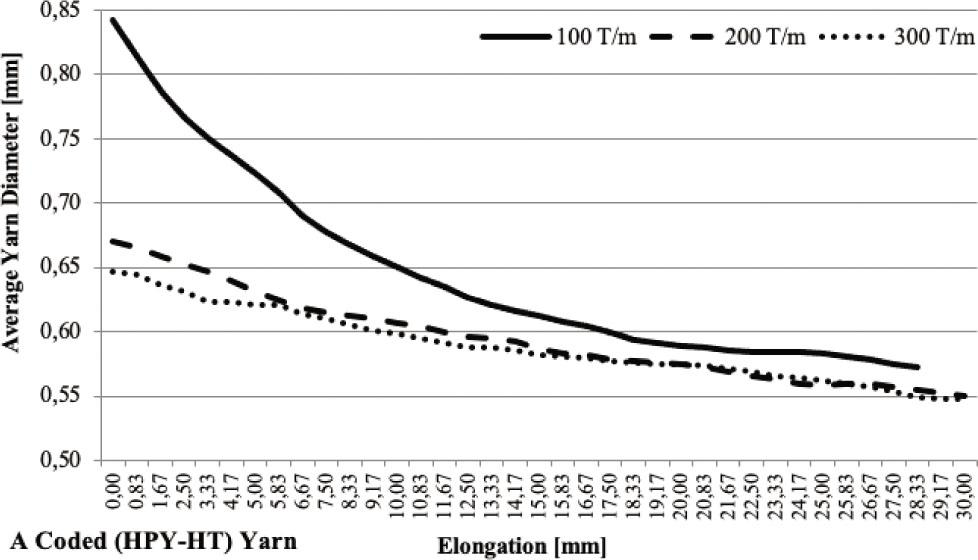Changes in the average yarn diameter of A-coded yarn depending on elongation.HPY, helical plied yarn; HT, high tenacity.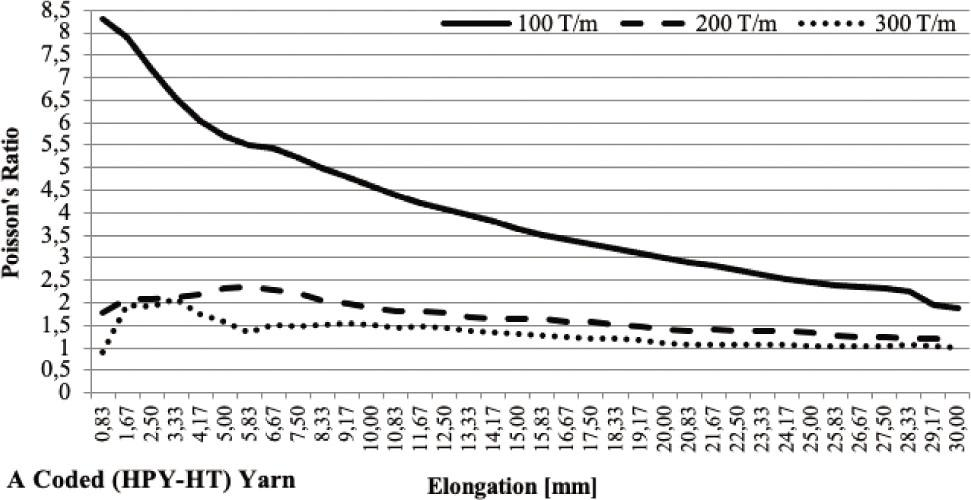Changes in the Poisson's ratio of A-coded yarn depending on elongation.HPY, helical plied yarn; HT, high tenacity.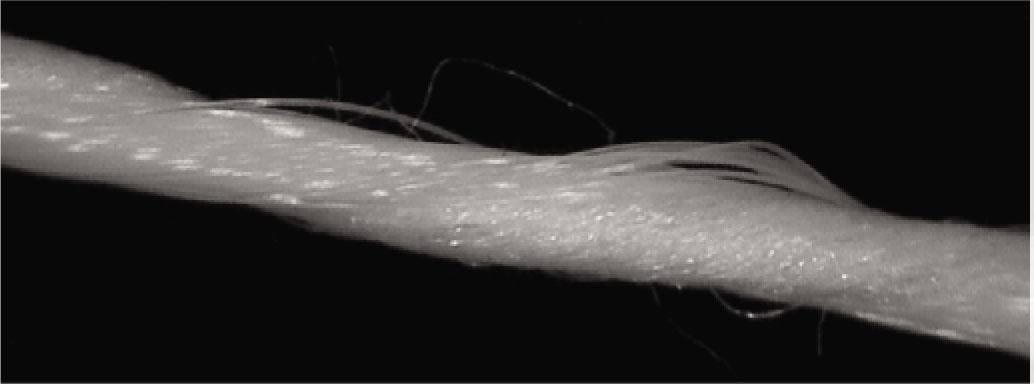A-coded yarn structure (HPY–HT) with 100 T/m.HPY, helical plied yarn; HT, high tenacity.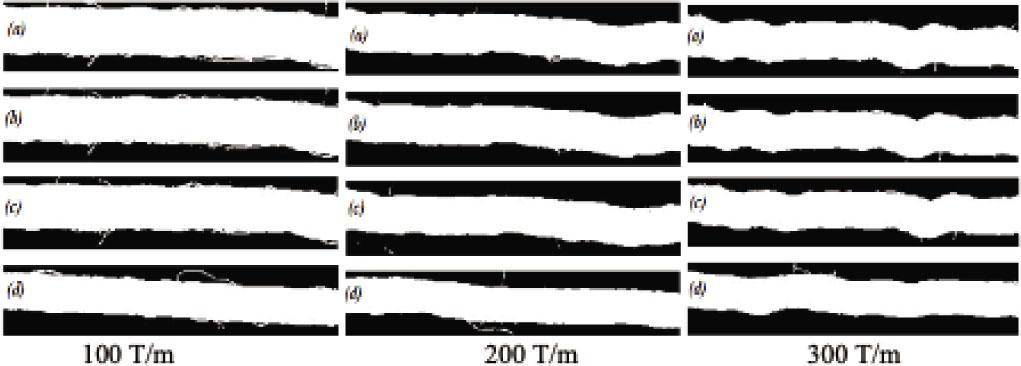A-coded yarn binary images: (a) tension-free state; (b) 5 sec; (c) 10 sec; (d) 60 sec.

In Figure 7, it was observed that the average yarn diameter of the A-coded yarn formed with HPY–HT decreased slightly linearly due to the tension. The changes in the average yarn diameters in yarns with 200 and 300 T/m twist levels were close to each other. Depending on the tension in the yarn with 100 T/m, the change in the yarn diameter was significant up to an elongation value of 10 mm, after which it had approximately the same tendency as the other yarns.

In Figure 8, when the Poisson's ratios of the yarns were examined, it was observed that the yarn produced with low twist level due to tension showed a significant change. The Poisson's ratios of the yarns produced with twist levels of 200 and 300 T/m were slightly decreased due to the tension.

Here, an NPR could not be obtained from a yarn structure produced by twisting together a bulky HPY yarn component and a stiff HT yarn. However, it was seen that the yarn twist values used in such a yarn structure have a significant effect on the Poisson's ratio. Although the results obtained did not yield an NPR for the HPY–HT plied yarn structure, it was observed that there was no significant change in the Poisson's ratios at high twist levels despite 30 mm elongation. This result showed that the HPY–HT plied yarn structure may have high stability at high elongation values.

Evaluation of the change in yarn diameter and Poisson's ratio of the B-coded yarn structure

The changes in the average yarn diameter and Poisson's ratio of the B-coded yarn (HPY–braid) depending on elongation are given in Figures 11 and 12. Moreover, the structure and binary images under different tension values of B-coded yarn produced at different twist levels are presented in Figures 13 and 14.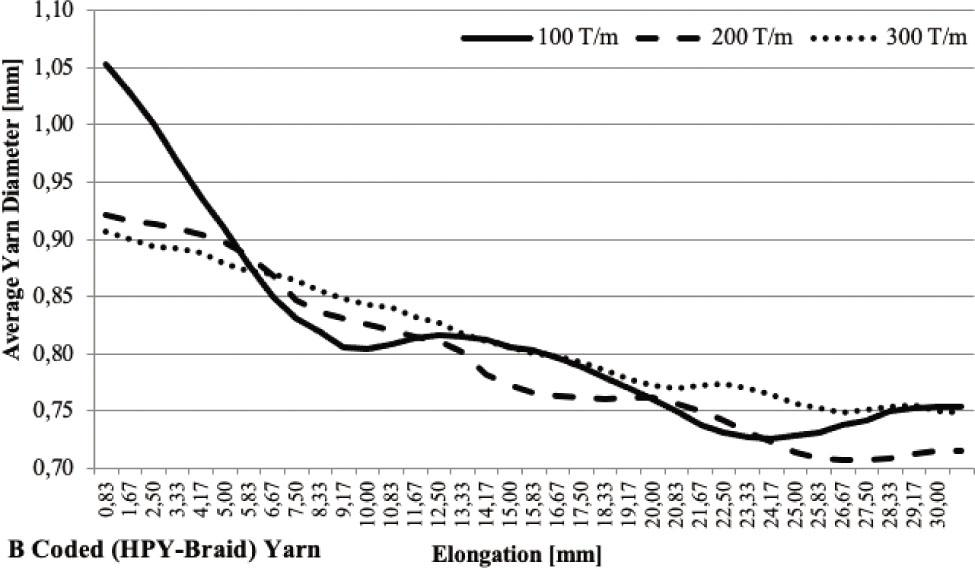Changes in the average yarn diameter of B-coded yarn depending on elongation.HPY, helical plied yarn.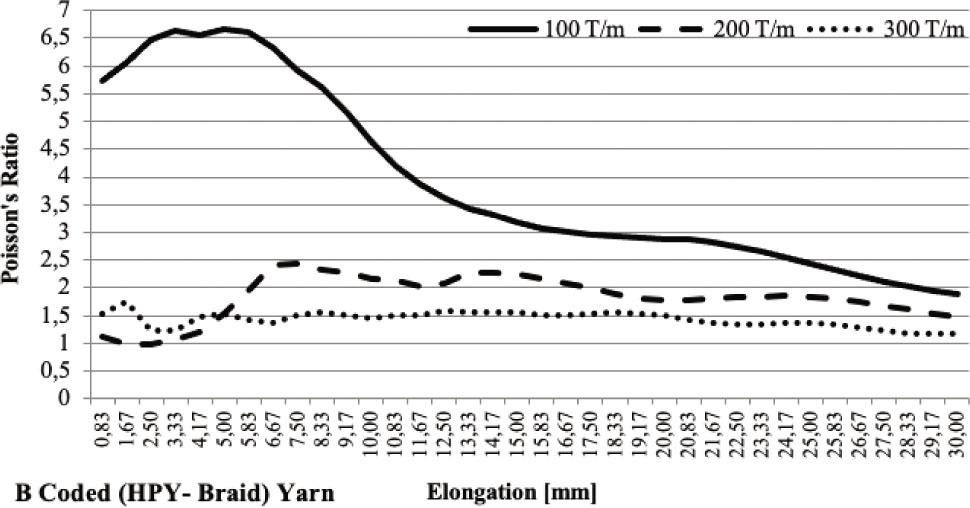Changes in the Poisson's ratio of B-coded yarn depending on elongation.HPY, helical plied yarn.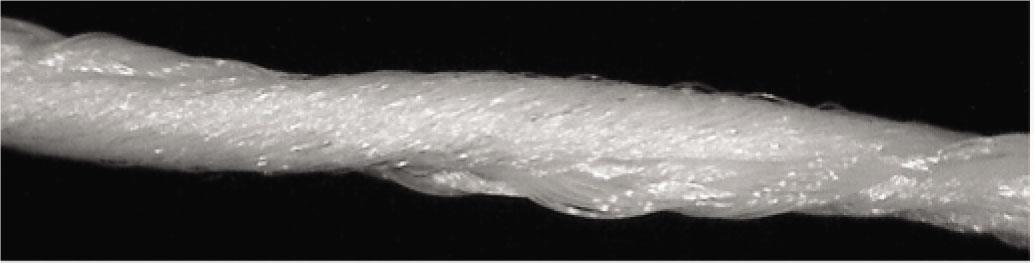B-coded yarn structure (HPY–braid) with 100 T/m.HPY, helical plied yarn.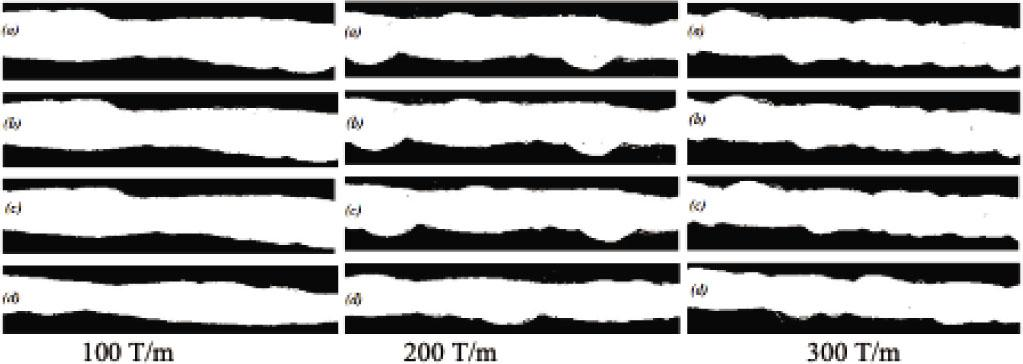B-coded yarn binary images: (a) tension-free state; (b) 5 sec; (c) 10 sec; (d) 60 sec.

In Figure 11, when the changes in the average yarn diameter of B-coded yarn were examined, fluctuating changes were observed in the average yarn diameter, unlike the changes of A-coded yarn. It was seen that these fluctuating changes in the yarn diameter were more pronounced in the yarn with a low twist level (100 T/m).

As a remarkable case in this study, it could be said that the yarns that have fluctuating changes in the average yarn diameter could yield partial NPRs under tension. Normally, Poisson's ratios are calculated by taking the difference between the initial diameter of the yarn and the diameter under tension (Eqs 1 and 2). Therefore, Poisson's ratios are obtained depending on the tensionless diameter value of the yarn. However, when the change of the yarn diameter was examined in Figure 11, it could be seen that for the 100 T/m yarn, the yarn diameter obtained at 9.17 mm (if the initial yarn diameter value was accepted) was lower than the yarn diameter obtained in the elongation range of 10.00–14.17 mm. Similarly, the yarn diameter obtained at 23.33 mm (if the initial yarn diameter value was accepted) was lower than the yarn diameter obtained in the elongation range of 24.17–30 mm. As a result of this, it could be said that the yarns that have a fluctuating change in average yarn diameter could yield partial NPRs under tension. The partial Poisson's ratios of 100 T/m twisted B-coded yarns are given in Table 5.

Partial Poisson's ratios of B-coded yarn.

Elongation (mm)Poisson's ratio
10.00−1.33065
10.83−1.43219
11.67−1.23961
12.50−0.80584
13.33−0.47961
14.17−0.05713
24.17−0.88423
25.00−1.04287
25.83−1.44305
26.67−1.47492
27.50−1.72514
28.33−1.61833
29.17−1.48626
30.00−1.26565

In Figure 12, when the changes in Poisson's ratios were examined, a positive Poisson's ratio was also obtained in the B-coded yarn structure. As observed in the A-coded yarn structure, it was found that the Poisson's ratios were low in high twist levels in the B-coded yarn structure. Unlike the A-coded yarn, the Poisson's ratio of B-coded yarn (HPY–braid) with 100 T/m was lower. It was observed that the Poisson's ratio of B-coded yarn structure at 300 T/m twist level remained stable, as was found in the A-coded yarn.

Evaluation of the change in yarn diameter and Poisson's ratios of the C-coded yarn structure

The changes in the average yarn diameter and Poisson's ratio of C-coded yarn (braid–braid) depending on elongation are given in Figures 15 and 16. In addition, the structure and binary images under different tension values of C-coded yarn produced at different twist levels are presented in Figures 17 and 18.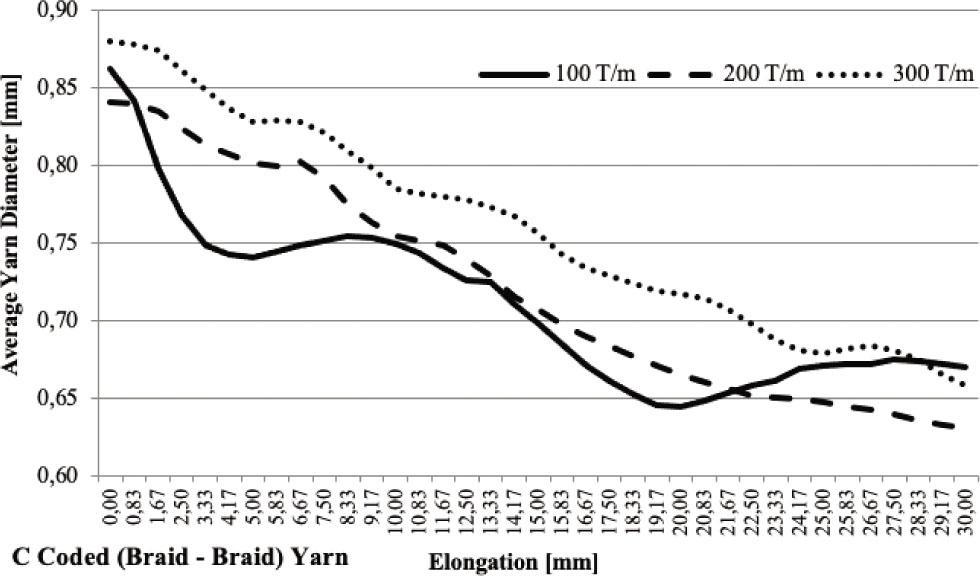Changes in the average yarn diameter of C-coded yarn depending on elongation.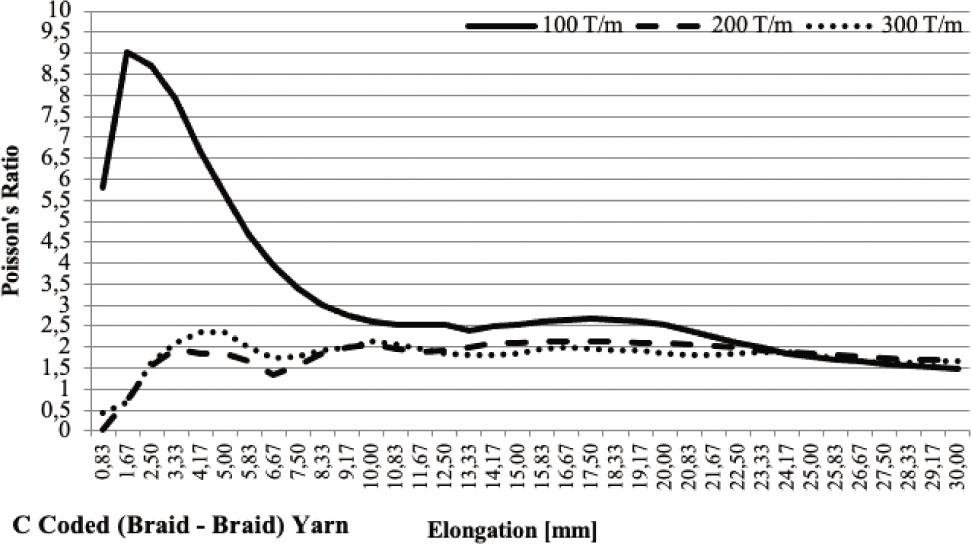Changes in the Poisson's ratio of C-coded yarn depending on elongation.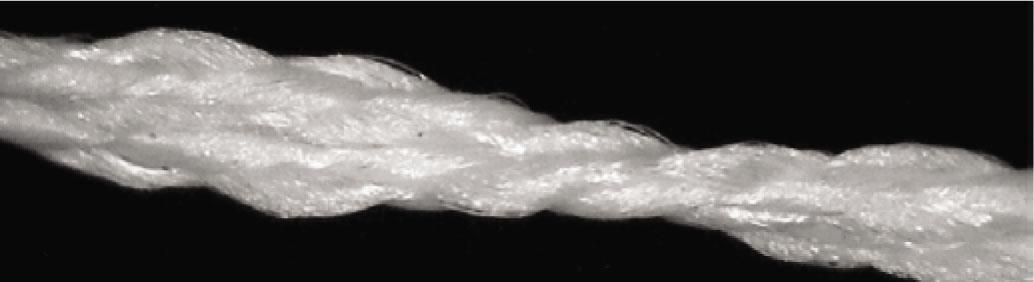C-coded yarn structure (braid–braid) with 100 T/m.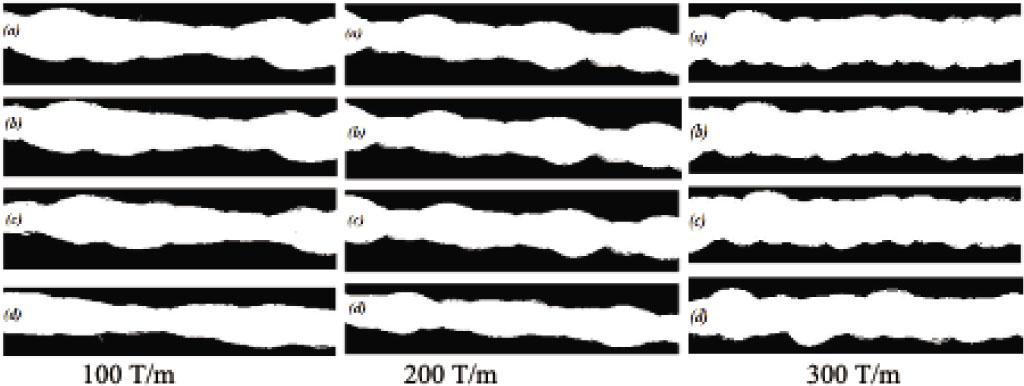C-coded yarn binary images: (a) tension-free state; (b) 5 sec; (c) 10 sec; (d) 60 sec.

In Figure 15, when the changes in the average yarn diameter of C-coded yarn were examined, a fluctuating change was observed, as was found in the B-coded yarn. This fluctuating change in yarn diameter was more pronounced in the yarn with a low twist level (100 T/m).

In Figure 15, when the change in yarn diameter was examined, it could be seen that for the 100 T/m yarn, the yarn diameter obtained at 5.00 mm (if the initial yarn diameter value was accepted) was lower than the yarn diameter obtained in the elongation range of 5.83–10.83 mm. Moreover, the yarn diameter obtained at 20.00 mm (if the initial yarn diameter value was accepted) was lower than the yarn diameter obtained in the elongation range of 20.83–30 mm. As a result of this, it could be said that the yarns that have fluctuating changes in the average yarn diameter could yield partial NPRs under tension. Partial Poisson's ratios of the 100 T/m twisted C-coded yarns are given in Table 6.

Partial Poisson's ratios of C-coded yarn.

Elongation (mm)Poisson's ratio
5.83−0.032866257
6.67−0.033303609
7.50−0.029697542
8.33−0.027346056
9.17−0.020560321
10.00−0.011593572
10.83−0.003736319
20.83−0.156943842
21.67−0.176623965
22.50−0.16818895
23.33−0.158297065
24.17−0.182503918
25.00−0.164179830
25.83−0.147433174
26.67−0.130384581
27.50−0.126287203
28.33−0.10919716
29.17−0.093590804
30.00−0.08137862

In Figure 16, when the changes in the Poisson's ratios of the C-coded yarn consisting of braid–braid yarn components were examined, it was seen that the 200 and 300 T/m twist levels were in the same trend. Furthermore, it was found that for these yarns (unlike the A and B yarns), Poisson's ratios approximately equaling zero could be obtained under approximately 1 mm elongation. High Poisson's ratios were obtained at low twist value, as seen in the A- and B-coded yarns. In the yarns with 100 T/m twist level, it was observed that after an elongation value of 10 mm, the Poisson's ratios approach those of the yarn structures with 200 and 300 T/m twist levels. In Figure 16, it was observed in the 200 and 300 T/m twisted yarn structure with the braid–braid yarn component that the Poisson's ratios, which were ≈ 0 in the 1 mm elongation state, increased positively due to the elongation. Besides, this increase did not rise to very high values and remained at a Poisson's ratio of about +2.

Evaluation of the change in yarn diameter and Poisson's ratios of the D-coded yarn structure

The changes in the average yarn diameter and Poisson's ratio of the D-coded yarn (HT–latex) depending on elongation are given in Figures 19 and 20. In addition, the structure and binary images under different tension values of D-coded yarn produced at different twist levels are presented in Figures 21 and 22. In Figure 19, when the changes in the average yarn diameter of the D-coded yarn were examined, a fluctuating change was observed, as was found in the B- and C-coded yarns. It was seen that this fluctuating change in yarn diameter was more pronounced in yarns with a low twist level (100 T/m).

In Figure 19, when the change of yarn diameter was examined, for the 100 T/m yarn, the yarn diameter obtained at 5.83 mm (if the initial yarn diameter value was accepted) was lower than the yarn diameter obtained in the elongation range of 6.67–16.67 mm. Moreover, the yarn diameter obtained at 18.33 mm (if the initial yarn diameter value was accepted) was lower than the yarn diameter obtained in the elongation range of 19.17–27.50 mm. Partial Poisson's ratios of the 100 T/m twisted C-coded yarns are given in Table 7.

Partial Poisson's ratios of D-coded yarn.

Elongation (mm)Poisson's ratio
6.67−0.023286305
7.50−0.036927530
8.33−0.038658832
9.17−0.03880524
10.00−0.035975603
10.83−0.031188364
11.67−0.024890606
12.50−0.019386194
13.33−0.014878261
14.17−0.010359011
15.00−0.006833548
15.83−0.003656841
16.67−0.001673751
19.17−0.009302206
20.00−0.007530813
20.83−0.014028275
21.67−0.024807405
22.50−0.029990742
23.33−0.034406199
24.17−0.035407814
25.00−0.036076492
25.83−0.032057647
26.67−0.020665704
27.50−0.008678707

In Figure 20, when the changes in the Poisson‘s ratios of the yarn consisting of HT–latex components were examined, an NPR was obtained in the range of 7.50–13.33 mm elongation for the yarn with 100 T/m. Besides, it was seen that the Poisson's ratios remained at quite a low level (range: ≈ 0.0–0.5) at all twist levels of the yarn structure formed by these components along the 30 mm extension distance. It was observed that the change in Poisson's ratio due to tension was lower in a D-coded yarn structure with latex–HT compound than the yarn structures coded A, B, and C.

Evaluation of the change in yarn diameter and Poisson's ratios of the E-coded yarn structure

The changes in the average yarn diameter and Poisson's ratio of the E-coded yarn (latex–PVC) depending on elongation are given in Figures 23 and 24. Moreover, the structure and binary images under different tension values of the E-coded yarn produced at different twist levels are presented in Figures 25 and 26..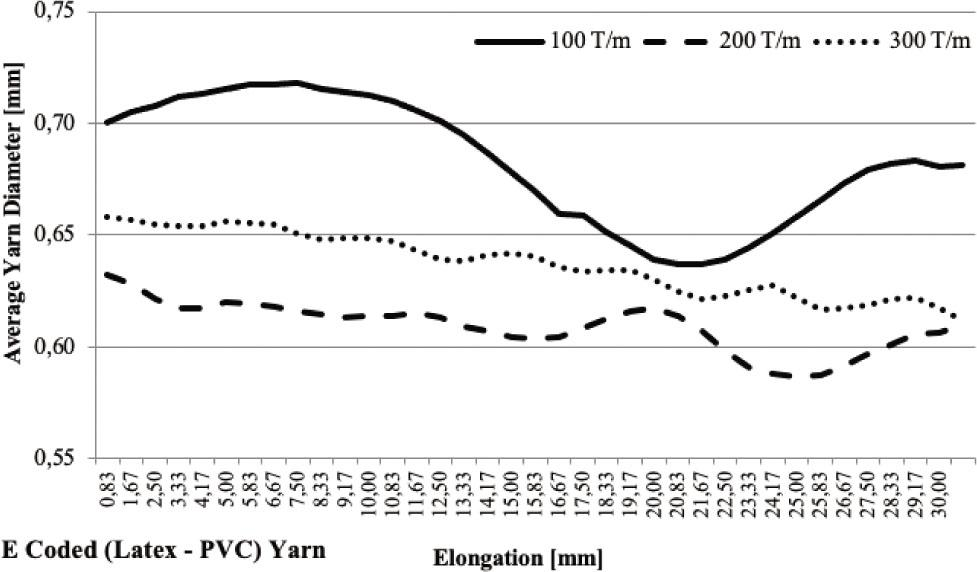Changes in the average yarn diameter of E-coded yarn depending on elongation.PVC, polyvinyl chloride.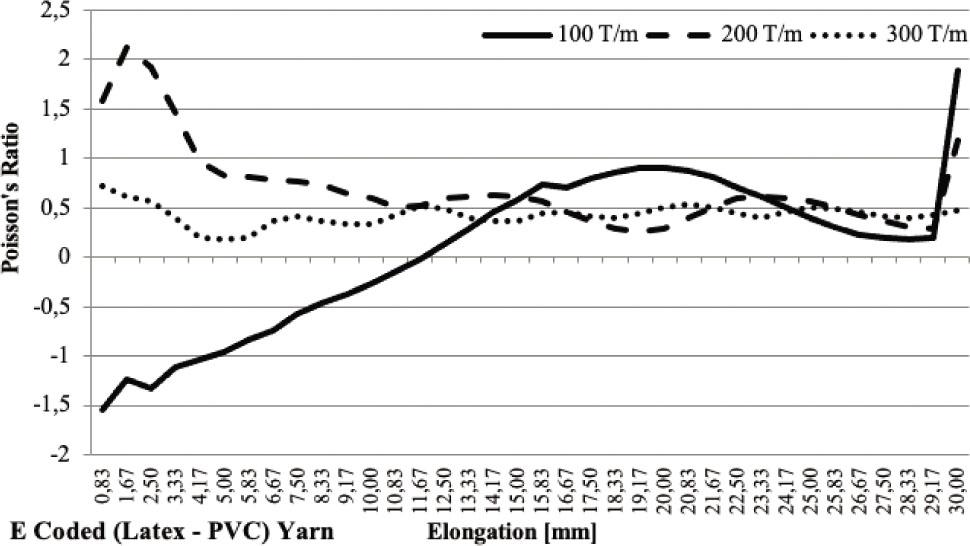Changes in the Poisson's ratio of E-coded yarn depending on elongation.PVC, polyvinyl chloride.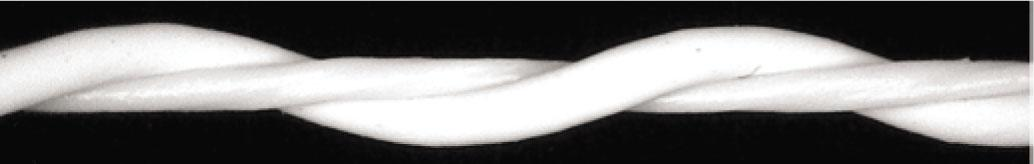E-coded yarn structure (latex–PVC) with 100 T/m.PVC, polyvinyl chloride.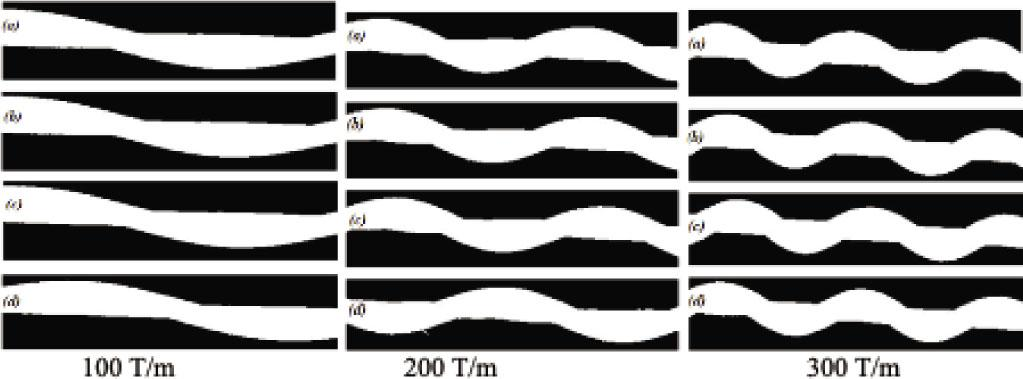E-coded yarn binary images: (a) tension-free state; (b) 5 sec; (c) 10 sec; (d) 60 sec.

In Figure 23, when the changes in the average yarn diameter of E-coded yarn were examined, a fluctuating change was observed, as was found in the B-, C-, and D-coded yarns. It was seen that this fluctuating change in yarn diameter was more pronounced in the yarn with a low twist level (100 T/m). The E-coded yarn gave NPRs from the beginning up to 11.67 mm elongation value (Figure 24). When the partial Poisson's ratios were examined (Figure 23), it could be seen that for the 100 T/m yarn, the yarn diameter obtained at 20.83 mm (if the initial yarn diameter value was accepted) was lower than the yarn diameter obtained in the elongation range of 21.67–30.00 mm. Partial Poisson's ratios of the 100 T/m twisted C-coded yarn are given in Table 8.

Partial Poisson's ratios of E-coded yarn.

Elongation (mm)Poisson's ratio
21.67−0.066820902
22.50−0.142437175
23.33−0.172753655
24.17−0.205634724
25.00−0.225858607
25.83−0.235102859
26.67−0.236735582
27.50−0.218538067
28.33−0.200092689
29.17−0.171274066
30.00−0.156218374

In Figure 24, when the changes in the Poisson's ratios of the yarn consisting of latex–PVC yarn components were analyzed, it was seen that the 100 T/m twisted yarn gave NPRs from the beginning up to 11.67 mm elongation value. Although the same result was observed in the yarn where the D-coded latex yarn component was used, an NPR was obtained after a certain tension in the D-coded yarn. In the E-coded yarn structure, the combination of the PVC-coated polyester yarn component, which had a harder structure than the HT yarn component used in the D yarn, with the latex yarn could have caused this result. Moreover, it was seen that the 100 T/m twisted yarn with latex–PVC yarn component gave a very high NPR (≈−1.5).

Evaluation of the change in yarn diameter and Poisson's ratios of the F-coded yarn structure

The changes in the average yarn diameter and Poisson's ratio of the F-coded yarn (braid–PVC) depending on elongation are given in Figures 27 and 28. Moreover, the structure and binary images under different tension values of the F-coded yarn produced at different twist levels are presented in Figures 29 and 30.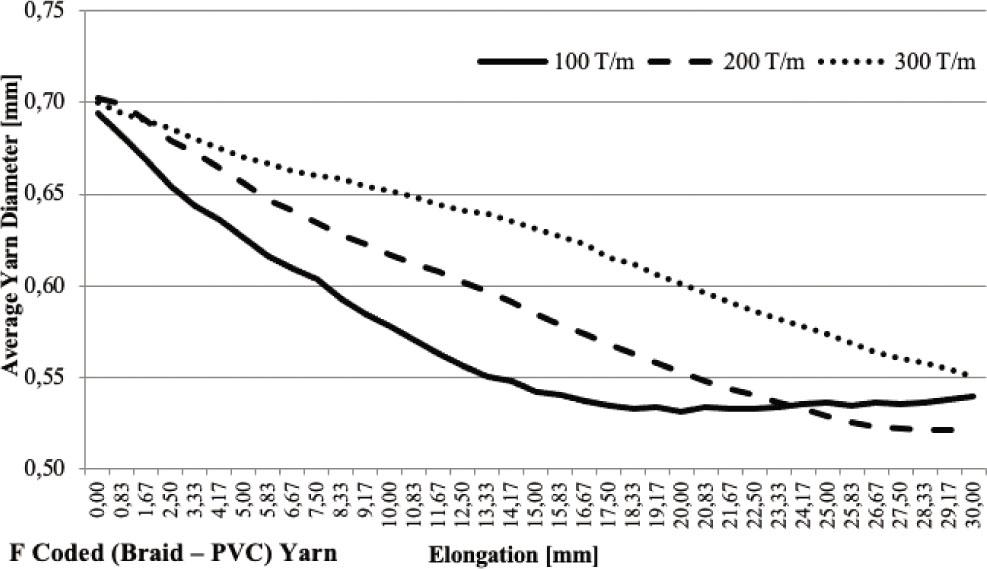Changes in the average yarn diameter of F-coded yarn depending on elongation.PVC, polyvinyl chloride.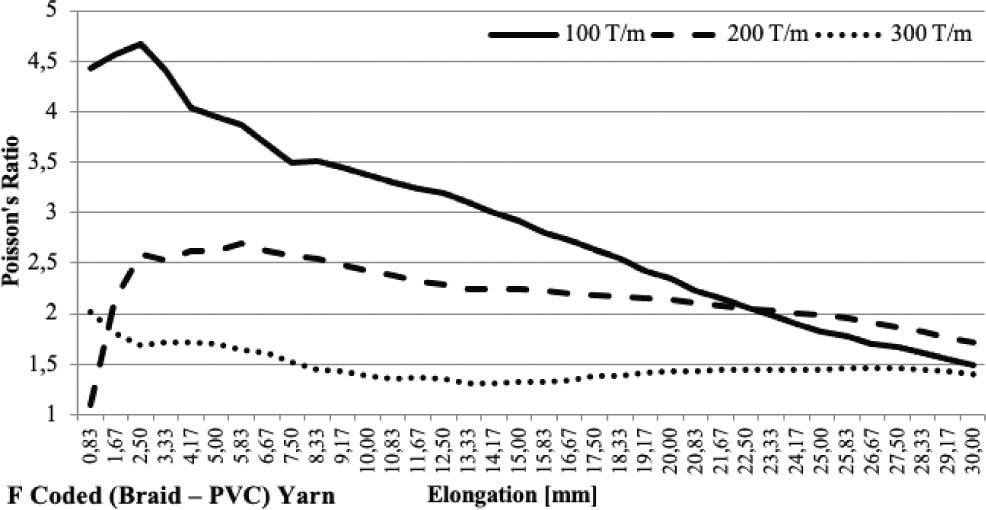Changes in the Poisson's ratio of F-coded yarn depending on elongation.PVC, polyvinyl chloride.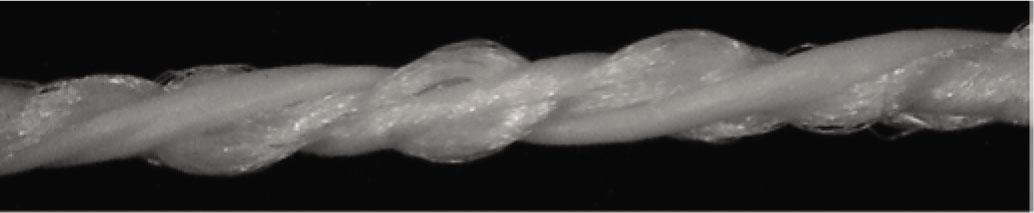F-coded yarn structure (braid–PVC) with 100 T/m.PVC, polyvinyl chloride.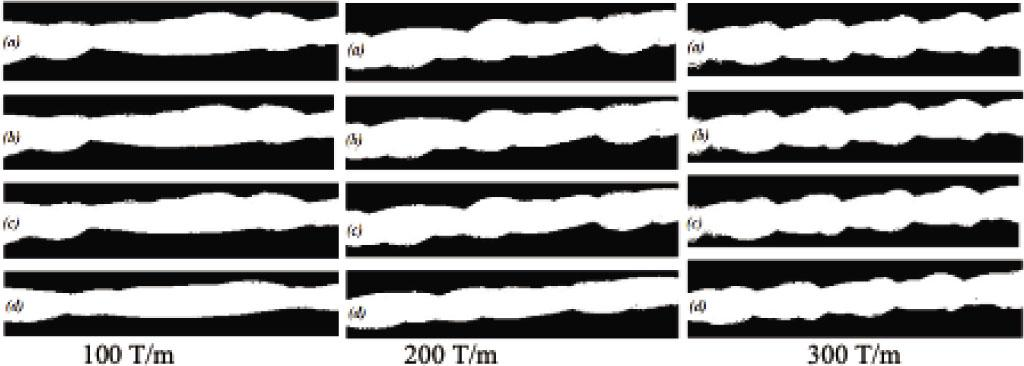F-coded yarn binary images: (a) tension-free state; (b) 5 sec; (c) 10 sec; (d) 60 sec.

In Figure 27, it was observed that the average yarn diameter of the F-coded yarn formed with the braid–PVC had a linear decreasing slope due to the tension, as was found in the A-coded (HPY–HT) yarn.

In Figure 28, when the Poisson's ratios of the yarn consisting of braid–PVC components were examined, positive Poisson's ratios were obtained. The most stable yarn structure was observed for the 300 T/m twisted yarn. There was no significant change in the Poisson's ratios due to tension in this yarn. The most significant change in Poisson's ratio was seen in the 100 T/m twisted yarn. It was observed that an NPR could not be obtained from the yarn structure formed by braid–PVC (bulky–stiff) components. However, it was observed that the Poisson's ratios of the braid–PVC plied yarn at a high twist level (300 T/m) remained approximately constant and low (≈ +1.5) under tensile loading.

Evaluation of the change in yarn diameter and Poisson's ratios of the G-coded yarn structure

The changes in the average yarn diameter and Poisson's ratio of the G-coded yarn (braid–latex) depending on elongation are given in Figures 31 and 32. Moreover, the structure and binary images under different tension values of the G-coded yarn produced at different twist levels are presented in Figures 33 and 34.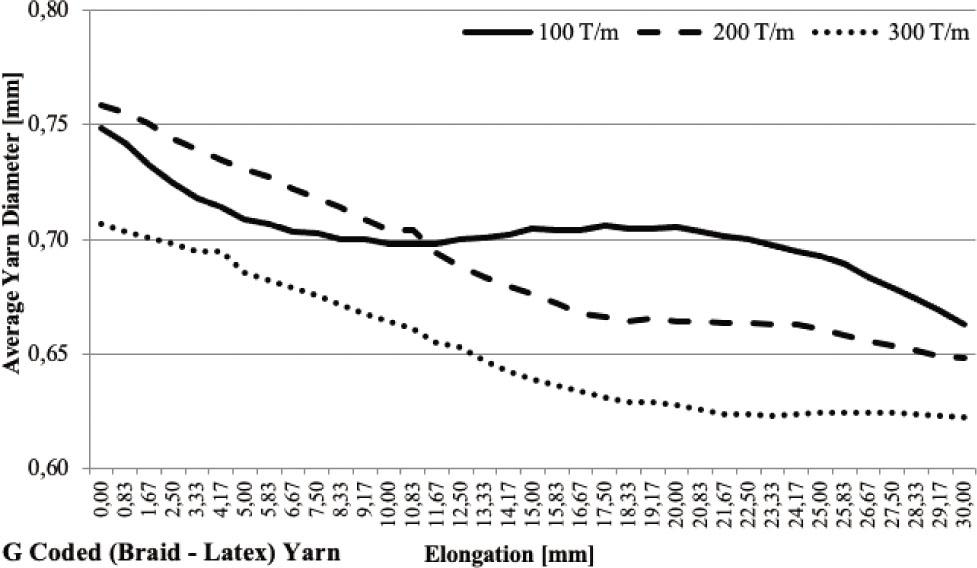Changes in the average yarn diameter of G-coded yarn depending on elongation.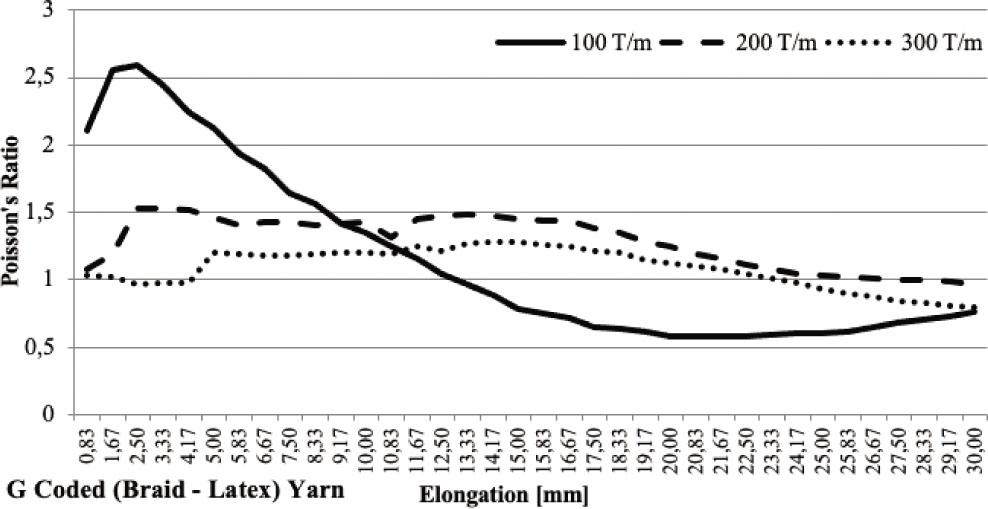Changes in the Poisson's ratio of G-coded yarn depending on elongation.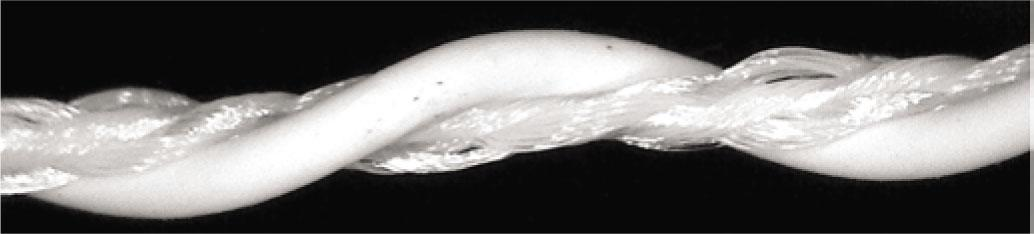G-coded yarn structure (braid–latex) with 100 T/m.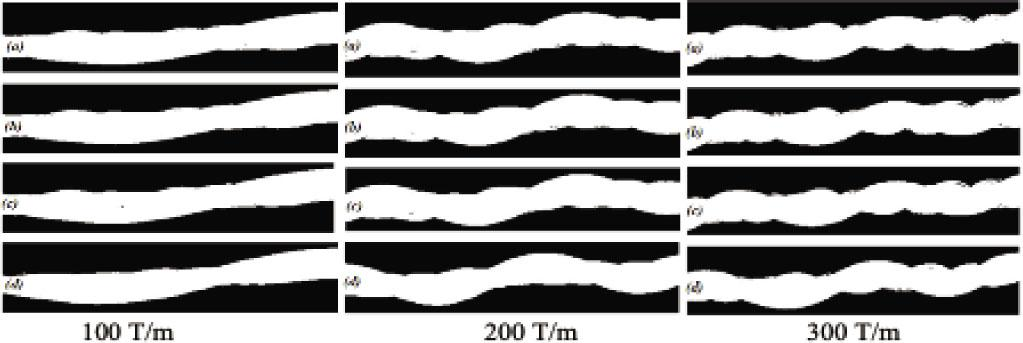G-coded yarn binary images: (a) tension-free state; (b) 5 sec; (c) 10 sec; (d) 60 sec.

In Figure 31, the average yarn diameter of the G-coded (braid–latex) yarn twisted with 200 and 300 T/m showed a linear decreasing slope due to the tension, as was observed in the A (HPY–HT) and F (braid–PVC)-coded yarns. It was observed that there was a slight fluctuation in the 100 T/m twisted yarn; this tendency first decreased, then remained approximately constant at the same level, and then gave decreasing change of yarn diameter values.

In Figure 32, when the Poisson's ratios of the G-coded yarn produced from braid–latex yarn components were examined, it was seen that stable Poisson's ratios were obtained at 200 and 300 T/m. It was also observed that the 200 and 300 T/m twisted yarns gave similar trends.

CONCLUSION

In this study, the change in Poisson's ratios of novel plied yarns consisting of different component yarn structures and with different twist levels were examined. The aim was to investigate the Poisson's ratios, as well as the possible auxetic effects, of novel plied yarn structures formed by bulky/soft (HPY, braid, and latex) and stiff (HT and PVC) yarn components.

The experimental results showed that as the twist level increased, the Poisson's ratios and the tenacity values of the novel plied yarns decreased, but the elongation values increased. NPR was observed in HT–latex and PVC–latex plied yarn with a low twist level.

As a remarkable case in this study, it could be said that the yarns that have fluctuating changes in the average yarn diameter could yield partial NPRs under tension. Results showed that the plied yarn structures consisting of braid–HPY and braid–braid component yarns gave a partial NPR effect under tension. A similar result was achieved in yarns with HT–latex and PVC–latex components. Since the partial NPR effect was observed in novel plied yarns with braid and HPY components, it was concluded that yarns formed with bulky–bulky yarn components could give an auxetic performance under tension. Experimental results showed that plied yarn structures with fluctuating yarn diameters under tension could give partial NPR and exhibit auxetic behavior under tension. It was also found that the fluctuating changes in yarn diameter were more pronounced in yarns with a low twist level.

The different structural architectural design of the yarns examined in the study is based on the auxetic behavior of plied structures formed by the combination of bulky yarn groups and rigid yarn groups. Besides, the effect of yarn twists on this plied yarn structure was evaluated. In this study, the NPR effect could be obtained from structures consisting of latex and rigid structured yarn components. In order to evaluate whether a structure consisting of bulky yarn components, such as braid and HPY, could exhibit an auxetic performance under tension, it was planned to examine the tension-dependent auxetic performances of the structure of plied yarns composed of bulky–rigid and bulky–bulky yarn components. It was tested whether the yarn structure consisting of braid and HPY could also expand in the transverse direction under tension. As a result of the study, it was observed that the structure composed of bulky yarn components, such as braid and HPY, exhibits partial NPR behavior under tension.

It could be envisaged that such plied yarns could be used in applications where they could exhibit intermittent (with a fluctuating) auxetic property up to a certain elongation stage after a certain tension level. For this purpose, it is proposed that research should be continued to produce braid and HPY yarn structures with different structural parameters to obtain partial NPR.

#### Partial Poisson's ratios of C-coded yarn.

Elongation (mm)Poisson's ratio
5.83−0.032866257
6.67−0.033303609
7.50−0.029697542
8.33−0.027346056
9.17−0.020560321
10.00−0.011593572
10.83−0.003736319
20.83−0.156943842
21.67−0.176623965
22.50−0.16818895
23.33−0.158297065
24.17−0.182503918
25.00−0.164179830
25.83−0.147433174
26.67−0.130384581
27.50−0.126287203
28.33−0.10919716
29.17−0.093590804
30.00−0.08137862

#### Tenacity and elongation values of component yarns.

Component yarnTenacity (N/tex)Maximum elongation (%)
HT0.6513.60
HPY0.3026.80
Braid0.3219.17
PVC0.1417.60

#### Properties of the novel plied yarn.

Yarn codeYarn count (tex)Yarn componentsYarn twist (T/m)Tenacity (N/tex)Maximum elongation (%)
A315.777HPY–HT1000.2913.66
2000.2837.02
3000.2842.77
B500.555HPY–braid1000.2827.76
2000.2836.92
3000.2536.50
C388.444Braid–braid1000.2918.39
2000.2823.03
3000.2626.53
D259.444HT–latex1000.02513.19
2000.02213.49
3000.02314.22
E361.111PVC–latex1000.04613.51
2000.04313.86
3000.04314.55
F305.333Braid–PVC1000.2214.92
2000.2318.35
3000.2022.91
G444.222Braid–latex1000.1318.93
2000.1220.64
3000.1224.47

#### Partial Poisson's ratios of B-coded yarn.

Elongation (mm)Poisson's ratio
10.00−1.33065
10.83−1.43219
11.67−1.23961
12.50−0.80584
13.33−0.47961
14.17−0.05713
24.17−0.88423
25.00−1.04287
25.83−1.44305
26.67−1.47492
27.50−1.72514
28.33−1.61833
29.17−1.48626
30.00−1.26565

#### Component yarn properties.

Component yarnYarn propertiesYarn count (tex)
HTHigh tenacity polyester9.444
HPYHelical plied yarn8 pieces of 33.333/96 tex/filament textured polyester yarn were produced by folding them by twisting at 70 T/m.306.333
BraidCircular braided yarn with 8 carriers.(4 × 33.333/96 tex/filament textured polyester + 4 × 9.444/36 tex/filament HT polyester)(The braided yarn was produced by attaching to the carrier in a 1:1 layout.)194.222
PVCPVC-coated polyester111.111
LatexMonofilament latex250.000

#### Correlation coefficients between twist level and tenacity, elongation, and Poisson's ratios of novel plied yarns.

Yarn codeCorrelation coefficients between twist level and tenacityCorrelation coefficients between twist level and elongationCorrelation coefficients between twist level and Poisson's ratio (at 10 mm elongation)
A (HPY–HT)−0.870.94−0.92
B (HPY–braid)−0.870.85−0.95
C (braid–braid)−0.981.00−0.87
D (HT–latex)−0.650.97
E (PVC–latex)−0.870.98
F (braid–PVC)−0.651.00−1.00
G (braid–latex)−0.870.98−0.63

#### Partial Poisson's ratios of E-coded yarn.

Elongation (mm)Poisson's ratio
21.67−0.066820902
22.50−0.142437175
23.33−0.172753655
24.17−0.205634724
25.00−0.225858607
25.83−0.235102859
26.67−0.236735582
27.50−0.218538067
28.33−0.200092689
29.17−0.171274066
30.00−0.156218374

#### Partial Poisson's ratios of D-coded yarn.

Elongation (mm)Poisson's ratio
6.67−0.023286305
7.50−0.036927530
8.33−0.038658832
9.17−0.03880524
10.00−0.035975603
10.83−0.031188364
11.67−0.024890606
12.50−0.019386194
13.33−0.014878261
14.17−0.010359011
15.00−0.006833548
15.83−0.003656841
16.67−0.001673751
19.17−0.009302206
20.00−0.007530813
20.83−0.014028275
21.67−0.024807405
22.50−0.029990742
23.33−0.034406199
24.17−0.035407814
25.00−0.036076492
25.83−0.032057647
26.67−0.020665704
27.50−0.008678707

Evans, K. E., Alderson, K. L. (2000). Auxetic materials: the positive side of being negative. Engineering Science and Education Journal, 9, 148–154.EvansK. E.AldersonK. L.2000Auxetic materials: the positive side of being negativeEngineering Science and Education Journal914815410.1049/esej:20000402Search in Google Scholar

Liu, Y., Hu, H. (2010). A review on auxetic structures and polymeric materials. Scientific Research and Essays, 5, 1052–1063.LiuY.HuH.2010A review on auxetic structures and polymeric materialsScientific Research and Essays510521063Search in Google Scholar

Miller, W., Hook, P. B., Smith, C. W., Wang, X., Evans, K. E. (2009). The manufacture and characterisation of a novel, low modulus, negative Poisson's ratio composite. Composites Science and Technology, 69(5), 651–655.MillerW.HookP. B.SmithC. W.WangX.EvansK. E.2009The manufacture and characterisation of a novel, low modulus, negative Poisson's ratio compositeComposites Science and Technology69565165510.1016/j.compscitech.2008.12.016Search in Google Scholar

Sloan, M. R., Wright, J. R., Evans, K. E. (2011). The helical auxetic yarn – A novel structure for composites and textiles; geometry, manufacture and mechanical properties. Mechanics of Materials, 43, 476–486.SloanM. R.WrightJ. R.EvansK. E.2011The helical auxetic yarn – A novel structure for composites and textiles; geometry, manufacture and mechanical propertiesMechanics of Materials4347648610.1016/j.mechmat.2011.05.003Search in Google Scholar

Wright, J. R., Burns, M. K., James, E., Sloan, M. R., Evans, K. E. (2012). On the design and characterisation of low-stiffness auxetic yarns and fabrics. Textile Research Journal, 82(7), 645–654.WrightJ. R.BurnsM. K.JamesE.SloanM. R.EvansK. E.2012On the design and characterisation of low-stiffness auxetic yarns and fabricsTextile Research Journal82764565410.1177/0040517512436824Search in Google Scholar

Du, Z. Q., Zhou, M., He, L. E., Liu, H. L. (2015). Study on negative Poisson's ratio of auxetic yarn under tension: Part 2 – experimental verification. Textile Research Journal, 85(7), 768–774.DuZ. Q.ZhouM.HeL. E.LiuH. L.2015Study on negative Poisson's ratio of auxetic yarn under tension: Part 2 – experimental verificationTextile Research Journal85776877410.1177/0040517514549987Search in Google Scholar

Bhattacharya, S., Zhang, G. H., Ghita, O., Evans, K. E. (2014). The variation in Poisson's ratio caused by interactions between core and wrap in helical composite auxetic yarns. Composites Science and Technology, 102, 87–93.BhattacharyaS.ZhangG. H.GhitaO.EvansK. E.2014The variation in Poisson's ratio caused by interactions between core and wrap in helical composite auxetic yarnsComposites Science and Technology102879310.1016/j.compscitech.2014.07.023Search in Google Scholar

Zhang, G. H., Ghita, O., Lin, C. P., Evans, K. E. (2016). Varying the performance of helical auxetic yarns by altering component properties and geometry. Composite Structures, 140, 369–377.ZhangG. H.GhitaO.LinC. P.EvansK. E.2016Varying the performance of helical auxetic yarns by altering component properties and geometryComposite Structures14036937710.1016/j.compstruct.2015.12.032Search in Google Scholar

Du, Z. Q., Zhou, M., Liu, H. L., He, L. E. (2015). Study on negative Poisson's ratio of auxetic yarn under tension: Part 1 - theoretical analysis. Textile Research Journal, 85(5), 487–498.DuZ. Q.ZhouM.LiuH. L.HeL. E.2015Study on negative Poisson's ratio of auxetic yarn under tension: Part 1 - theoretical analysisTextile Research Journal85548749810.1177/0040517514549985Search in Google Scholar

Lim, T. C. (2014). Semi-auxetic yarns. Physica Status Solidi B, 251(2), 273–280.LimT. C.2014Semi-auxetic yarnsPhysica Status Solidi B251227328010.1002/pssb.201384237Search in Google Scholar

Ge, Z., Hu, H., Liu, S. (2016). A novel plied yarn structure with negative Poisson's ratio. The Journal of the Textile Institute, 107(5), 578–588.GeZ.HuH.LiuS.2016A novel plied yarn structure with negative Poisson's ratioThe Journal of the Textile Institute107557858810.1080/00405000.2015.1049069Search in Google Scholar

Liu, S., Du, Z., Xie, K., Liu, G., Yang, S. (2018). A novel interlaced-helical wrapping yarn with negative Poisson's ratio. Fibers and Polymers, 19, 2411–2417.LiuS.DuZ.XieK.LiuG.YangS.2018A novel interlaced-helical wrapping yarn with negative Poisson's ratioFibers and Polymers192411241710.1007/s12221-018-8391-9Search in Google Scholar

Jiang, N., Hu, H. (2019). Auxetic yarn made with circular braiding technology. Physica Status Solidi B, 256, 1–12.JiangN.HuH.2019Auxetic yarn made with circular braiding technologyPhysica Status Solidi B25611210.1002/pssb.201800168Search in Google Scholar

Jiang, N., Hu, H. (2018). A study of tubular braided structure with negative Poisson's ratio behavior. Textile Research Journal, 88(24), 2810–2824.JiangN.HuH.2018A study of tubular braided structure with negative Poisson's ratio behaviorTextile Research Journal88242810282410.1177/0040517517732086Search in Google Scholar

• #### Modeling of Material Characteristics of Conventional Synthetic Fabrics

Artículos recomendados de Trend MD